Courses

# Formative Assessment- Linear Equations in One Variable Class 8 Notes | EduRev

## Mathematics (Maths) Class 8

Created by: Full Circle

## Class 8 : Formative Assessment- Linear Equations in One Variable Class 8 Notes | EduRev

The document Formative Assessment- Linear Equations in One Variable Class 8 Notes | EduRev is a part of the Class 8 Course Mathematics (Maths) Class 8.
All you need of Class 8 at this link: Class 8

Ques 1: Complete the following crossword puzzle using the given directions for across (from left to right) and down (from top to bottom):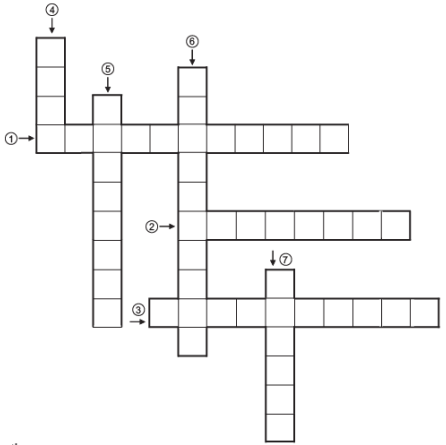Directions:

Accross:

(1) __________. means taking a term from one side of an equation to the other side with its sign changed.

(2) The value of the variable in an equation which makes LHS and RHS equal is called __________.

(3) In an algebraic term ax, ‘a’ is called __________of x.

Down:

(4) The other name of a solution of an equation.

(5) A literal symbol which takes on various numerical values is called a __________.

(6) A combination of constants and variables, connected by some or all basic operations is called an algebraic __________.

(7) A polynomial of degree 1 is called a __________ polynomial.

Ques 2: Match the following:

 Column A Column B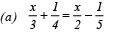( i ) x = –4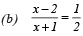(ii) x = –39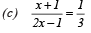(iii) x = 5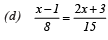(iv) x = 27/10

Ques 3: (i) Which of the following is not an equation:

(a)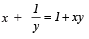(b)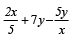(c)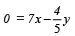(d) 2x – 3y + 7 = –1

(ii) Which of the following is a root of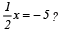(a) 10
(b) –10
(c) 0
(d)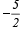(iii) Which of the following is the value of x in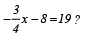(a)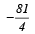(b) 81/4

(c) – 3 6
(d) 36

(iv) Which of the following is the value of x in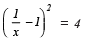(a) 1/3
(b)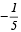(c)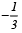(d) 1/5

Ques 4: (i) Is px2–qy = 1 a linear equation?

(ii)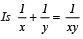a linear equation?

(iii) An equation which, when reduced to a simple form, involve no power of the variable quantity higher than ___________ is called a simple equation. Fill in the blank.
(iv) Which of these is the same as 9x + 7 = 8 ?

(a) 9x = 8/7
(b) 9x + 7–7 = 8–7
(c) 9x + 7–8 = 8–8
(d)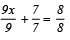(v) The equation 2x–1 = 5 is identical to:
(a) 3x – 1 = 5
(b) 4x – 2 = 10
(c) x = 2
(d) 4x + 2 = 10

1. (1) → TRANSPOSING
(2) → SOLUTION
(3) → COEFFICIENT
(4) → ROOT

(5) → VARIABLE
(6) → EXPRESSION
(7) → LINEAR

2. (a) → (iv)
(b) → (iii)
(c) → (i)
(d) → (ii)

3. (i) (b)
(ii) (b)
(iii) (c)
(iv) (a)

4. (i) No.
(ii) Yes
(iii) One
(iv) (b)
(v) (b)

,

,

,

,

,

,

,

,

,

,

,

,

,

,

,

,

,

,

,

,

,

;